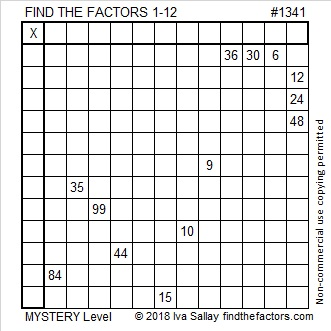# 1341 Not a Pretty Picture

In real mysteries, sometimes clues are close together, sometimes they are far apart. They don’t often make a pretty picture. Can you use the clues and logic to solve this mystery?Print the puzzles or type the solution in this excel file: 12 factors 1333-1341

Here is some information about the number 1341:

• 1341 is a composite number.
• Prime factorization: 1341 = 3 × 3 × 149, which can be written 1341 = 3² × 149
• 1341 has at least one exponent greater than 1 in its prime factorization so √1341 can be simplified. Taking the factor pair from the factor pair table below with the largest square number factor, we get √1341 = (√9)(√149) = 3√149
• The exponents in the prime factorization are 2 and 1. Adding one to each exponent and multiplying we get (2 + 1)(1 + 1) = 3 × 2 = 6. Therefore 1341 has exactly 6 factors.
• The factors of 1341 are outlined with their factor pairs in the graphic below.1341 is the sum of two squares:
30² + 21² = 1341

1341 is the hypotenuse of a Pythagorean triple:
459-1260-1341 which is 9 times (51-140-149) and
can also be calculated from 30² – 21², 2(30)(21), 30² + 21²

This site uses Akismet to reduce spam. Learn how your comment data is processed.